# Inverse Trigonometric Functions Class NCERT Solutions

## In this pdf file you can see answers of following Questions

### EXERCISE 2.1

Question 1. Find the principal values of the following: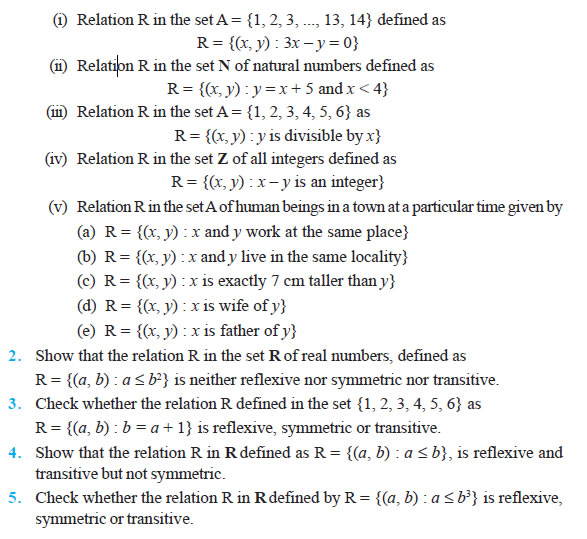Question 6. Show that the relation R in the set {1, 2, 3} given by R = {(1, 2), (2, 1)} is symmetric but neither reflexive nor transitive.

Question 7. Show that the relation R in the set A of all the books in a library of a college, given by R = {(x, y) : x and y have same number of pages} is an equivalence relation.

Question 8. Show that the relation R in the set A = {1, 2, 3, 4, 5} given by R = {(a, b) :
|a – b| is even}, is an equivalence relation. Show that all the elements of {1, 3, 5} are related to each other and all the elements of {2, 4} are related to each other. But no element of {1, 3, 5} is related to any element of {2, 4}.

Question 9. Show that each of the relation R in the set A = {x ∈ Z : 0 ≤ x ≤ 12}, given by (i) R = {(a, b) : |a – b| is a multiple of 4} (ii) R = {(a, b) : a = b} is an equivalence relation. Find the set of all elements related to 1 in each case.

Question 10. Give an example of a relation. Which is
(i) Symmetric but neither reflexive nor transitive.
(ii) Transitive but neither reflexive nor symmetric.
(iii) Reflexive and symmetric but not transitive.
(iv) Reflexive and transitive but not symmetric.
(v) Symmetric and transitive but not reflexive.

Question 11. Show that the relation R in the set A of points in a plane given by R = {(P, Q) : distance of the point P from the origin is same as the distance of the point Q from the origin}, is an equivalence relation. Further, show that the set of all points related to a point P ≠ (0, 0) is the circle passing through P with origin as centre.

Question 12. Show that the relation R defined in the set A of all triangles as R = {(T1, T2) : T1 is similar to T2}, is equivalence relation. Consider three right angle triangles T1 with sides 3, 4, 5, T2 with sides 5, 12, 13 and T3 with sides 6, 8, 10. Which triangles among T1, T2 and T3 are related?

Question 13. Show that the relation R defined in the set A of all polygons as R = {(P1, P2) : P1 and P2 have same number of sides}, is an equivalence relation. What is the set of all elements in A related to the right angle triangle T with sides 3, 4 and 5?

Question 14. Let L be the set of all lines in XY plane and R be the relation in L defined as R = {(L1, L2) : L1 is parallel to L2}. Show that R is an equivalence relation. Find the set of all lines related to the line y = 2x + 4.

Question 15. Let R be the relation in the set {1, 2, 3, 4} given by R = {(1, 2), (2, 2), (1, 1), (4,4), (1, 3), (3, 3), (3, 2)}. Choose the correct answer.
(A) R is reflexive and symmetric but not transitive.
(B) R is reflexive and transitive but not symmetric.
(C) R is symmetric and transitive but not reflexive.
(D) R is an equivalence relation.

Question 16.
Let R be the relation in the set N given by R = {(a, b) : a = b – 2, b > 6}. Choose the correct answer.
(A) (2, 4) ∈ R
(B) (3, 8) ∈ R
(C) (6, 8) ∈ R
(D) (8, 7) ∈ R

### EXERCISE 1.2

Question 1. Show that the function f : R → R defined by f (x) = 1 x is one-one and onto, where R is the set of all non-zero real numbers. Is the result true, if the domain R is replaced by N with co-domain being same as R?

Question 2. Check the injectivity and surjectivity of the following functions:
(i) f : N → N given by f (x) = x2
(ii) f : Z → Z given by f (x) = x2
(iii) f : R → R given by f (x) = x2
(iv) f : N → N given by f (x) = x3
(v) f : Z → Z given by f (x) = x3

Question 3. Prove that the Greatest Integer Function f :
R→R, given by f (x) = [x], is neither one-one nor onto, where [x] denotes the greatest integer less than or equal to x.

Question 4. Show that the Modulus Function f : R→R, given by f (x) = | x |, is neither oneone nor onto, where | x | is x, if x is positive or 0 and | x | is – x, if x is negative. 5. Show that the Signum Function f : R→R, given by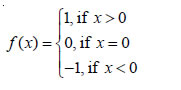is neither one-one nor onto.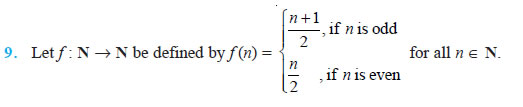Question 10. Let A = R – {3} and B = R – {1}. Consider the function f : A → B defined by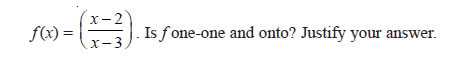Question 11. Let f : R → R be defined as f(x) = x4. Choose the correct answer.
(A) f is one-one onto (B) f is many-one onto
(C) f is one-one but not onto
(D) f is neither one-one nor onto.

Question 12. Let f : R → R be defined as f (x) = 3x. Choose the correct answer.
(A) f is one-one onto
(B) f is many-one onto
(C) f is one-one but not onto
(D) f is neither one-one nor onto.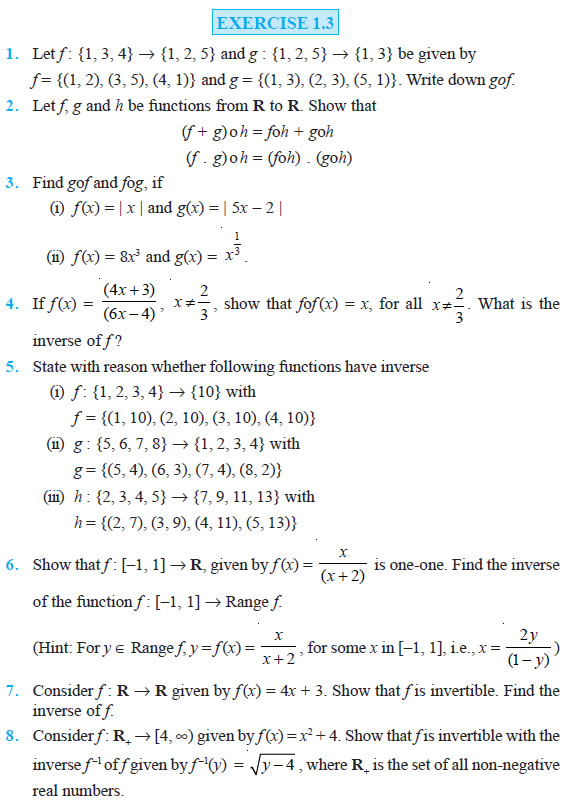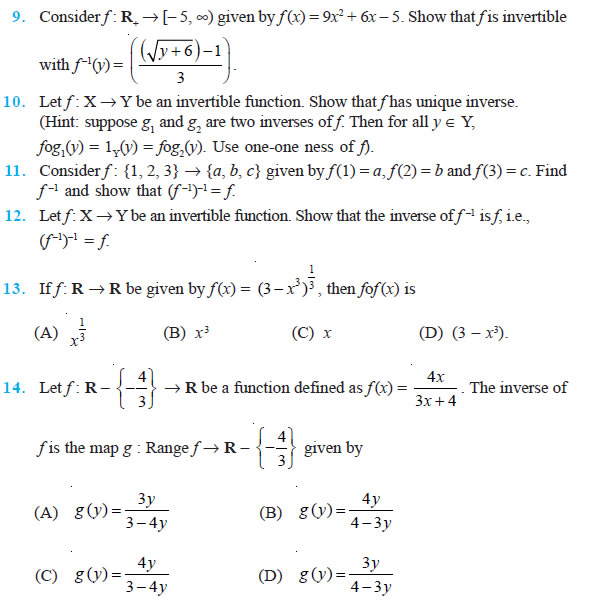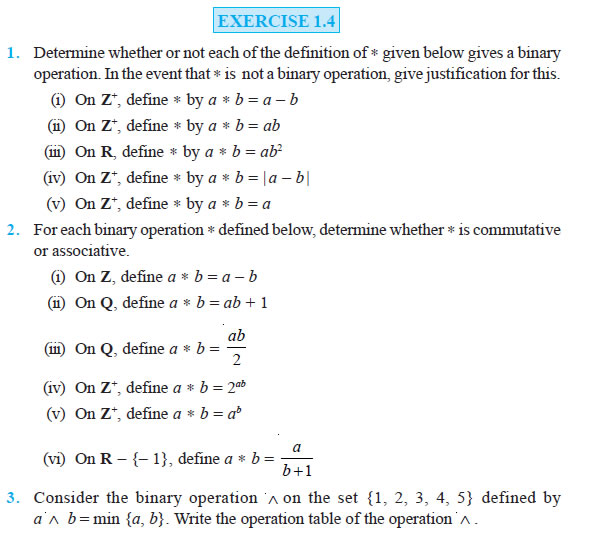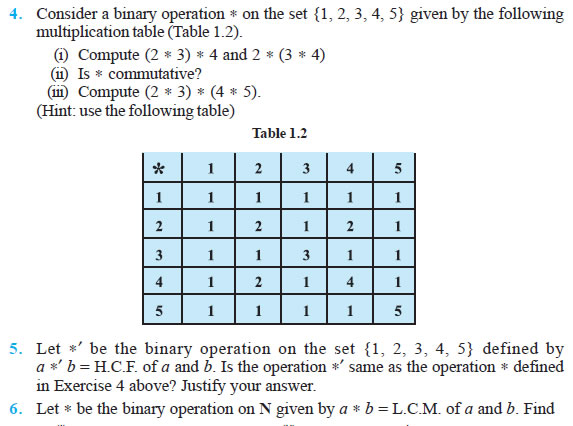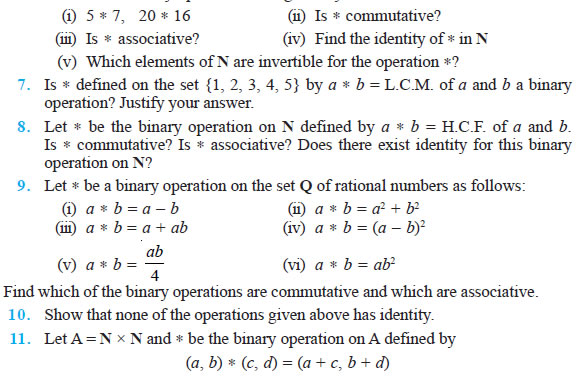Show that is commutative and associative. Find the identity element for on A, if any.

Question 12. State whether the following statements are true or false. Justify.
(i) For an arbitrary binary operation on a set N, a a = a ∀ a ∈ N.
(ii) If is a commutative binary operation on N, then a (b c) = (c b) a

Question 13. Consider a binary operation on N defined as a b = a3 + b3. Choose the correct answer.
(A) Is both associative and commutative?
(B) Is commutative but not associative?
(C) Is associative but not commutative?
(D) Is neither commutative nor associative?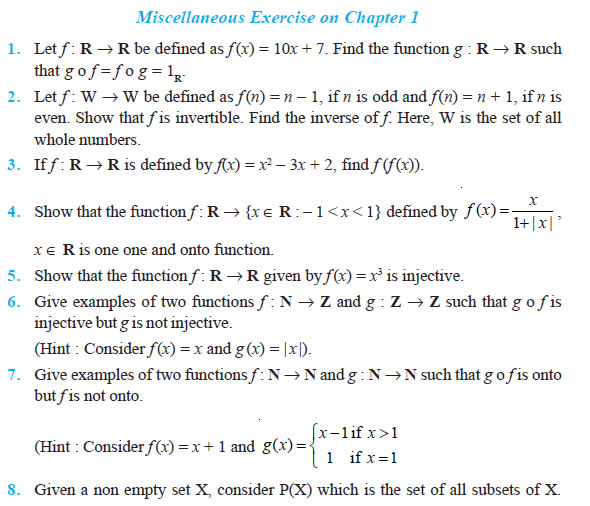Define the relation R in P(X) as follows: For subsets A, B in P(X), ARB if and only if A ⊂ B. Is R an equivalence relation on P(X)? Justify your answer.

Question 9. Given a non-empty set X, consider the binary operation :
P(X) × P(X) → P(X) given by A B = A ∩ B ∀ A, B in P(X), where P(X) is the power set of X. Show that X is the identity element for this operation and X is the only invertible element in P(X) with respect to the operation

Question 10. Find the number of all onto functions from the set {1, 2, 3, ... , n} to itself.

Question 11. Let S = {a, b, c} and T = {1, 2, 3}. Find F–1 of the following functions F from S to T, if it exists.
(i) F = {(a, 3), (b, 2), (c, 1)}
(ii) F = {(a, 2), (b, 1), (c, 1)}

Question 12. Consider the binary operations : R × R → R and o : R × R → R defined as a b = |a – b| and a o b = a, ∀ a, b ∈ R. Show that is commutative but not associative, o is associative but not commutative. Further, show that ∀ a, b, c ∈ R, a (b o c) = (a b) o (a b). [If it is so, we say that the operation distributes over the operation o]. Does o distribute over ? Justify your answer.

Question 13. Given a non-empty set X, let : P(X) × P(X) → P(X) be defined as A * B = (A – B) ∪ (B – A), ∀ A, B ∈ P(X). Show that the empty set φ is the identity for the operation and all the elements A of P(X) are invertible with A–1 = A. (Hint : (A – φ) ∪ (φ – A) = A and (A – A) ∪ (A – A) = A A = φ).

Question 14. Define a binary operation on the set {0, 1, 2, 3, 4, 5} as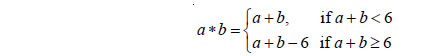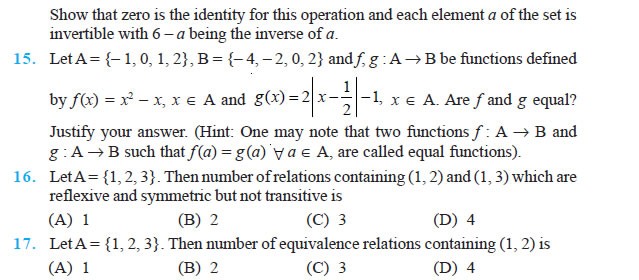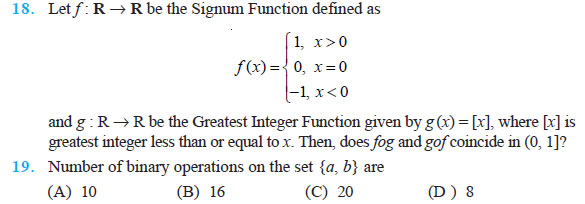### NCERT Books Free Pdf Download for Class 5, 6, 7, 8, 9, 10 , 11, 12 Hindi and English Medium

 Mathematics Biology Psychology Chemistry English Economics Sociology Hindi Business Studies Geography Science Political Science Statistics Physics Accountancy